Name:    Chemical Reactions/Stoichiometry Practice Test

Multiple Choice
Identify the choice that best completes the statement or answers the question.

1.

A(n) ____ chemical equation has the same number of atoms of each element on each side of the equation.
 a. unbalanced b. complex c. simple d. balanced

2.

What is the whole number that appears in front of a formula in a chemical equation?
 a. a subscript b. a coefficient c. a ratio d. a superscript

3.

Which equation is not balanced?
 a. 2H2 + O2  ®   H2O c. 4H2 + 2O2 ® 4H2O b. 2H2 + O2 ® 2H2O d. H2 + H2 + O2 ® H2O + H2O

4.

The coefficients in a chemical equation represent the
 a. number of each atom in each compound in a reaction. b. relative numbers of moles of reactants and products. c. number of valence electrons involved in the reaction. d. masses, in grams, of all reactants and products.

5.

Each substance to the right of the arrow in a chemical equation is a(n) ____.
 a. reactant b. catalyst c. inhibitor d. product

6.

Which observations indicate that a chemical reaction has occurred?
 a. production of a gas c. all of the above b. formation of a precipitate d. evolution of heat and light

7.

In writing a chemical equation that produces hydrogen gas, the correct representation of hydrogen gas is
 a. OH. b. H. c. 2H. d. H2.

8.

According to the law of conservation of mass, the total mass of the reacting substances is
 a. sometimes more and sometimes less than the total mass of the products. b. always less than the total mass of the products. c. always equal to the total mass of the products. d. always more than the total mass of the products.

9.

In what kind of reaction do two or more substances combine to form a new compound?
 a. ionic reaction c. synthesis reaction b. double-replacement reaction d. decomposition reaction

10.

The reaction, performed in lab, represented by the equation:
Zn(s) + 2HCl(aq) ® H2­ + ZnCl2(aq) is a
 a. decomposition reaction. c. double-replacement reaction. b. single-replacement reaction. d. composition reaction.

11.

The reaction, performed in lab, represented by the equation:
2Mg(s) + O2(g) ® 2MgO(s) is a
 a. decomposition reaction. c. double-replacement reaction. b. single-replacement reaction. d. synthesis reaction.

12.

The reaction, performed in lab, represented by the equation:
C25H52 + 38O2 ® 25CO2  + 26H2O is a(n)
 a. synthesis reaction. c. decomposition reaction. b. single-replacement reaction. d. combustion reaction.

13.

The reaction, performed in lab, represented by the equation:
Pb(NO3)2(aq) + 2NaI(aq) ® PbI2¯ + 2NaNO3(aq) is a
 a. double-replacement reaction. c. decomposition reaction. b. combustion reaction. d. synthesis reaction.

14.

The reaction, performed in lab, represented by the equation:
2H2O2(aq)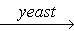2H2O(l) + O2­ is a(n)
 a. single-replacement reaction. c. decomposition reaction. b. double-replacement reaction. d. synthesis reaction.

15.

What are the correct coefficients when this equation is balanced?
___K  + ___Br2 ® ___KBr
 a. 2, 1, 2 b. 2, 1, 1 c. 1, 1, 1 d. 1, 2, 1

16.

What are the correct coefficients when this equation is balanced?
___Sb  +  ___O2  ® ___Sb4O6
 a. 10, 5, 1 b. 1, 2, 10 c. 4, 6, 1 d. 4, 3, 1

17.

Which word equation represents the reaction that produces water from hydrogen and oxygen?
 a. Water can be separated into hydrogen and oxygen. b. Water is decomposed from hydrogen and oxygen. c. H2 + O2 ® water. d. Hydrogen plus oxygen yields water.

18.

Which of the following is the correct skeleton equation for the reaction that takes place when solid phosphorus combines with oxygen gas to form diphosphorus pentoxide?
 a. P(s)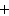O2(g) ® P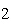O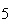(s) c. PO(s) ® P(s)O(g) b. P(s)O(g) ® PO(g) d. P(s)O(g) ® PO(g)

19.

Rewrite the following word equation as a balanced chemical equation. What is the coefficient and symbol for fluorine?
nitrogen trifluoride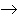nitrogenfluorine
 a. 6F b. F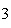c. 6Fd. 3F20.

What do you call a solid substance formed from mixing two aqueous solutions like shown in this figure?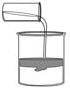a. catalyst b. synthesis c. aqueous d. precipitate

21.

In an equation, the symbol for a substance dissolved in water solution is followed by
 a. (aq). b. (1). c. (s). d. (g).

22.

What doesmean?
 a. A catalyst is needed in the reaction. b. Heat is supplied to the reaction. c. Electricity is need in the reaction. d. A precipitate will form during the reaction.

23.

A chemical formula written over the arrow in a chemical equation signifies
 a. an impurity. c. the formation of a gas. b. a catalyst for the reaction. d. a by-product.

24.

A catalyst is
 a. the product of a combustion reaction. b. speeds up but not used up in a reaction. c. a solid product of a reaction. d. one of the reactants in single-replacement reactions.

25.

A balanced chemical equation allows one to determine the
 a. electron configuration of all elements in the reaction. b. energy released in the reaction. c. mechanism involved in the reaction. d. mole ratio of any two substances in the reaction.

26.

In the reaction represented by the equation N2 + 3H2 ® 2NH3, what is the mole ratio of hydrogen to ammonia?
 a. 3:2 b. 1:1 c. 2:1 d. 6:8

27.

For the reaction represented by the equation C + 2H2 ® CH4, how many moles of hydrogen are required to produce 1 mol of methane, CH4? (look at equation)
 a. 4 mol b. 0.5 mol c. 2 mol d. 1 mol

28.

For the reaction represented by the equation 2H2 + O2 ® 2H2O, how many moles of water can be produced from 6.0 mol of oxygen? (look at equation)
 a. 12 mol b. 2.0 mol c. 6.0 mol d. 18 mol

29.

What is the first step in most stoichiometry problems?
 a. convert given quantities to moles b. convert given quantities to masses c. add the coefficients of the reagents d. convert given quantities to volumes

30.

For the reaction represented by the equation 2Na + 2H2O ® 2NaOH + H2, how many grams of hydrogen are produced from 80. g of water? (3 steps)
 a. 200 g b. 45 g c. 4.5 g d. 80. g

31.

For the reaction represented by the equation 2H2 + O2 ® 2H2O, how many grams of water are produced from 134.4 L of hydrogen at STP? (3 steps)
 a. 108 g b. 54.0 g c. 2.00 g d. 6.00 g

32.

For the reaction represented by the equation CH4 + 2O2 ® CO2 + 2H2O, how many moleculess of carbon dioxide are produced from the combustion of 100. g of methane?
 a.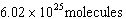c. 37.6 moles b.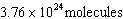d. 100 molecules

33.

A chemical reaction involving substances A and B stops when B is completely used. B is the
 a. primary product. c. limiting reactant. b. excess reactant. d. primary reactant.

34.

Which reactant controls the amount of product formed in a chemical reaction?
 a. excess reactant c. composition reactant b. limiting reactant d. mole ratio

35.

Which statement is true if 12 mol CO and 12 mol FeOare allowed to react?
3CO(g) + FeO(s)2Fe(s) + 3CO(g)
 a. The limiting reagent is CO and 8.0 mol Fe will be formed. b. The limiting reagent is CO and 3.0 mol COwill be formed. c. The limiting reagent is FeOand 24 mol Fe will be formed. d. The limiting reagent is FeOand 36 mol COwill be formed.